# Unified Council - UIMO PDF Sample Papers for Class 2

Resources:

Class 2 sample paper & practice questions for Unified International Mathematics Olympiad (UIMO) level 1 are given below. Syllabus for level 1 is also mentioned for these exams. You can refer these sample paper & quiz for preparing for the UIMO exam.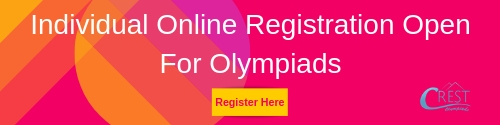Q.1 Q.2 Q.3 Q.4 Q.5 Q.6 Q.7 Q.8 Q.9 Q.10
 Q.1 Find the missing weight to balance the scale.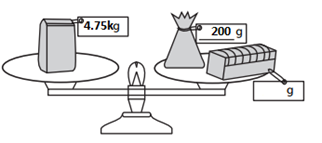a) 3550 kg b) 455 kg c) 4.55 kg d) 4550 kg
 Q.2 Find the odd one out.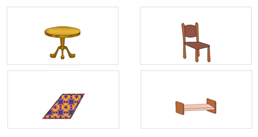a) 1 b) 2 c) 3 d) 4
 Q.3 Find the remainder if we take away 95 from 499. a) 404 b) 594 c) 425 d) 415
 Q.4 There are four more girls than boys in a class. There are 26 students in all. How many boys are there in the class? a) 11 b) 8 c) 9 d) 7
 Q.5 How many different numbers are shown below?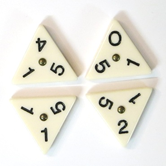a) 4 b) 6 c) 5 d) 12
 Q.6 Which is the odd one out? a)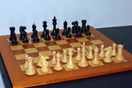b)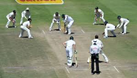c)d)Q.7 Meena's grandfather is 79 years old. Her father is 26 years younger than her grandfather. What is her father's age? a) 59 b) 56 c) 53 d) 63
 Q.8 Which three numbers among the following options have a sum of 789? a) 70 + 80 + 9 b) 700 + 800 + 9 c) 699 + 79 + 11 d) 700 + 89 + 9
 Q.9 Find the middle of the ruler.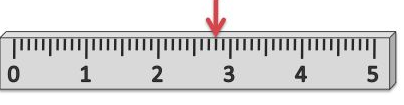a) Exactly below the red arrow b) To the left of the red arrow c) To the right of the red arrow d) Can not find
 Q.10 The cost of one red box is Rs. 100, one blue box is Rs. 50, and one green box is Rs. 20. Find the total cost of the boxes given below.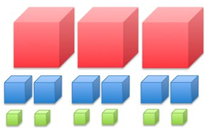a) Rs. 580 b) Rs. 640 c) Rs. 720 d) Rs. 880Sample PDF of Unified Council - Unified International Mathematics Olympiad (UIMO) PDF Sample Papers for Class 2: